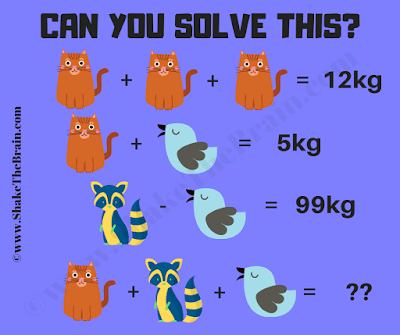This is a simple math brain teaser for school going students. Solving this math puzzle will test your mathematical skills. In this math brain teaser, you are shown some algebraic equations. Solve these simultaneous equations to find the value of each variable and then solve the last equation. So can you solve this and find the value of the missing number?Can you solve this Math Brain Teaser?
Answer of this "Math Brain Teaser", can be viewed by clicking on the answer button.